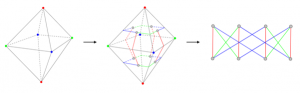Triangulations are a way of decomposing surfaces into triangles. For a variety of purposes, it is of interest to understand what the “average” triangulation looks like. A uniform sampling procedure for triangulations provides a way of doing so. In this blog post, we go over how a triangulation can be encoded as a network with coloured arcs, which in turn can be encoded as a pair of permutations. Thus, triangulations can be sampled by sampling pairs of permutations. We finish by providing some brief reasoning on why a uniform sample from the space of permutation pairs does not correspond to a uniform sample from the space of triangulations.

Surfaces are an important object in mathematics, and triangulations are a way of decomposing surfaces into triangles. Picture the octahedron—it’s an example of a triangulation of the sphere with eight triangles.

When we say “uniform sampling procedure for triangulations”, what we mean is: for a given number of triangles, how can we come up with a way of sampling from the space of triangulations that contain that many triangles? Now, you may be able to find a way of doing this by sampling coordinates in 3-dimensional space. However, we want to think about abstract triangulations; ones that aren’t embedded in a coordinate system. How do we even go about sampling such objects?

Well, there is a way to uniquely encode a triangulation as something known as a graph-encoded manifold or GEM. The diagram below shows how this can be achieved on the octahedron.First, we colour the vertices using three colours so that each triangle has a different colour on each vertex. Then, treating each triangle as a grey node, we place an arc between each pair of triangles that share a common edge. These arcs are coloured according to the single colour that was not used by the two vertices adjacent to the edge that the arc crosses. Finally, we “lift” the nodes and arcs off the octahedron and lay them down so that the red arcs are vertical. This is the GEM.

Great, so hopefully now it’s a little clearer what we mean by “sampling triangulations”—we’re actually sampling these networks, or combinatorial isomorphism types for those readers who understand what that means.

However, we’re still not completely clear on how to sample these GEMs! Well, see how in the previous diagram we arranged the nodes so that they formed two sets of four? That was on purpose—it allows us to represent a GEM using a pair of permutations. If we number the nodes and treat the green arcs as a mapping from the top nodes to the bottom nodes, it is easy to see how this represents a permutation. A similar thing can be done for the blue arcs. The diagram below shows what these two permutations are, written in cycle notation.By construction of the GEM, the red arcs will always represent the identity permutation, so we only need two permutations,  and , to represent the GEM.

Hooray! We’ve boiled down our problem of sampling triangulations to sampling pairs of permutations. However, a uniform sample from the space of permutation pairs does NOT correspond to a uniform sample from the space of triangulations. Why is that? Spoiler: while there is a one-to-one correspondence between triangulations and GEMs, this is NOT the case between GEMs and pairs of permutations.

In our report, we deal with issues such as disconnected GEMs, isomorphisms and symmetries to arrive at a procedure that does, in fact, sample triangulations uniformly.

Rajan Shankar
The University of SydneyView Rajan Shankar
Profile